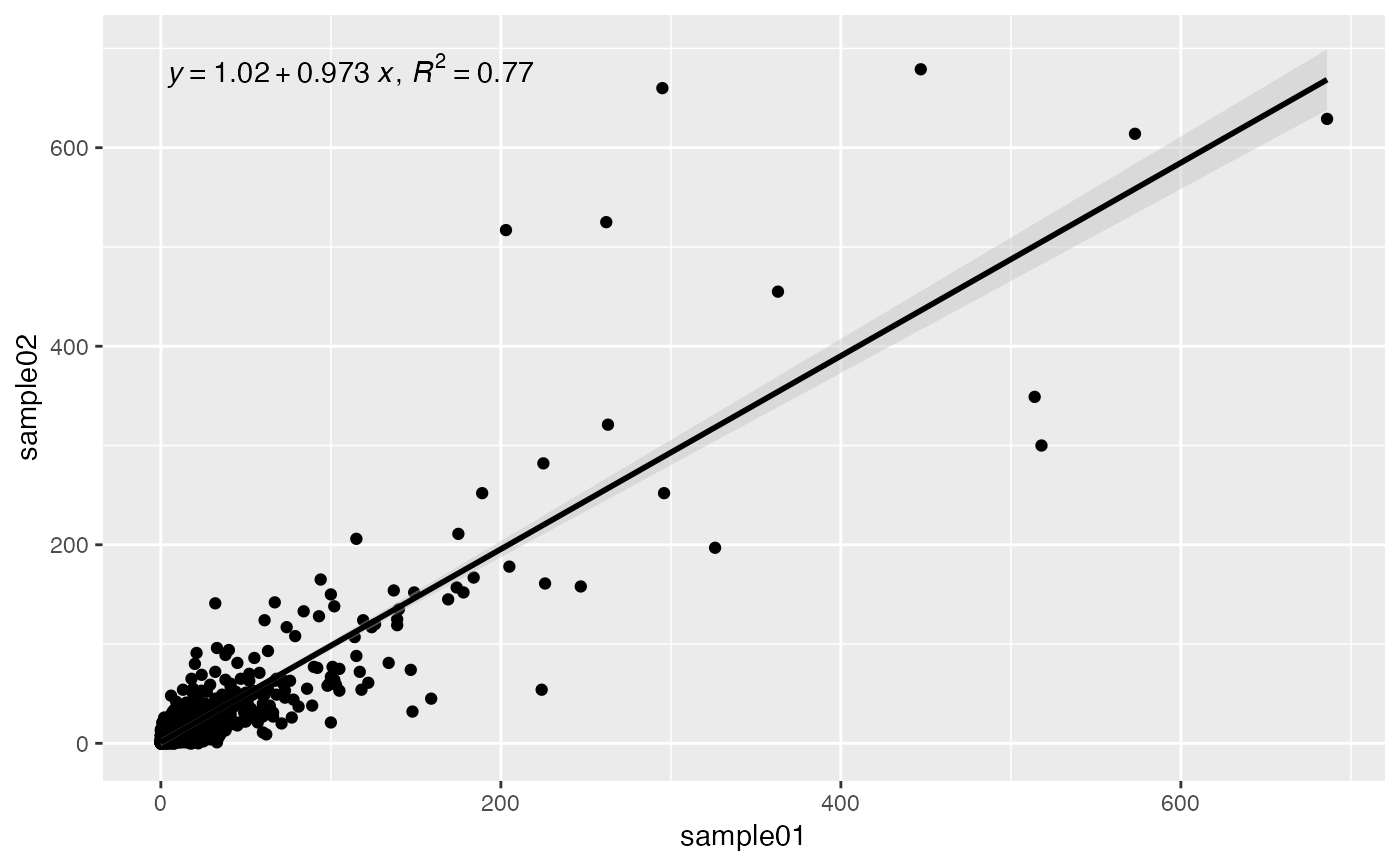Correlation X-Y scatterplot

## Usage

plotCorrelation(object, ...)

# S4 method for DFrame
plotCorrelation(object, labelCol = NULL, ...)

# S4 method for Matrix
plotCorrelation(
object,
xCol,
yCol,
label = FALSE,
labels = NULL,
trans = c("log10", "log2", "identity"),
r2 = TRUE,
se = TRUE,
colors = list(dots = "black", line = "black", se = "gray")
)

# S4 method for SummarizedExperiment
plotCorrelation(object, assay = 1L, ...)

# S4 method for data.frame
plotCorrelation(object, labelCol = NULL, ...)

# S4 method for matrix
plotCorrelation(
object,
xCol,
yCol,
label = FALSE,
labels = NULL,
trans = c("log10", "log2", "identity"),
r2 = TRUE,
se = TRUE,
colors = list(dots = "black", line = "black", se = "gray")
)

## Arguments

object

Object.

labelCol

character(1) or NULL. For data.frame method, which column should be used to label points on the plot?

...

xCol, yCol

character(1) or integer(1). X and Y column name or position.

label

logical(1). Superimpose sample text labels on the plot.

labels

list. ggplot2 labels. See ggplot2::labs() for details.

trans

character(1). Name of the axis scale transformation to apply.

help(topic = "scale_x_continuous", package = "ggplot2")

r2

logical(1). Show information on the lm fit? This includes the equation, and the coefficient of determination (R^2). Refer to ggpmisc::stat_poly_eq for details.

se

logical(1). Display confidence interval around the lm fit line? Refer to ggplot2::geom_smooth for details.

colors

list(3). Named list defining the colors of dots, line, and se, for confidence interval standard error.

assay

vector(1). Assay name or index position.

Plot.

## Note

Updated 2023-08-11.

## Correlation coefficient calculations

Correlation coefficient calcluations are generated by ggpmisc::stat_poly_eq(). Refer to the ggpmisc GitHub repo for details.

• ggpmisc::stat_poly_eq.

• ggplot2::geom_smooth.

## Examples

data(RangedSummarizedExperiment, package = "AcidTest")

## SummarizedExperiment ====
object <- RangedSummarizedExperiment
plotCorrelation(
object = object,
xCol = 1L,
yCol = 2L,
trans = "identity"
)